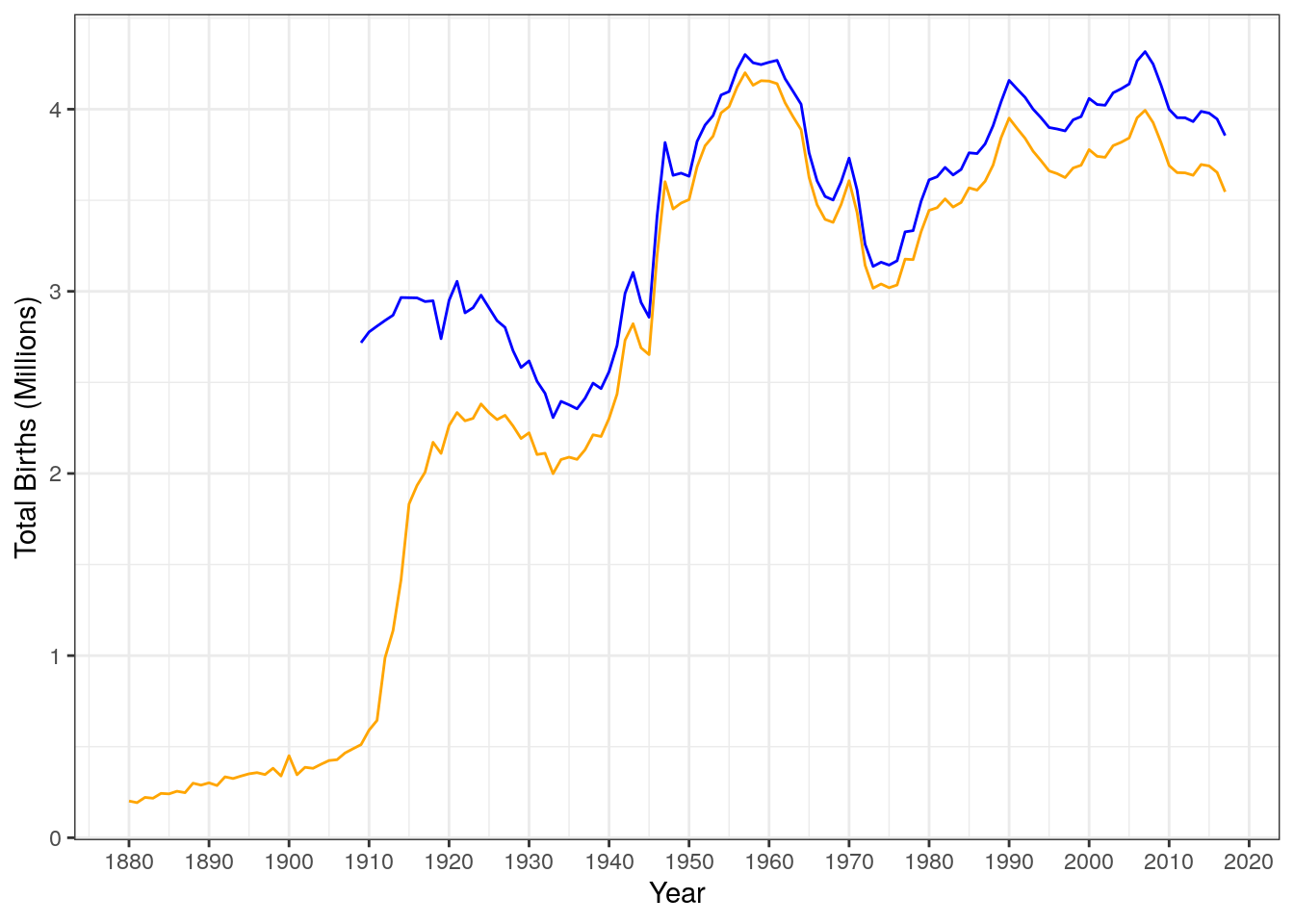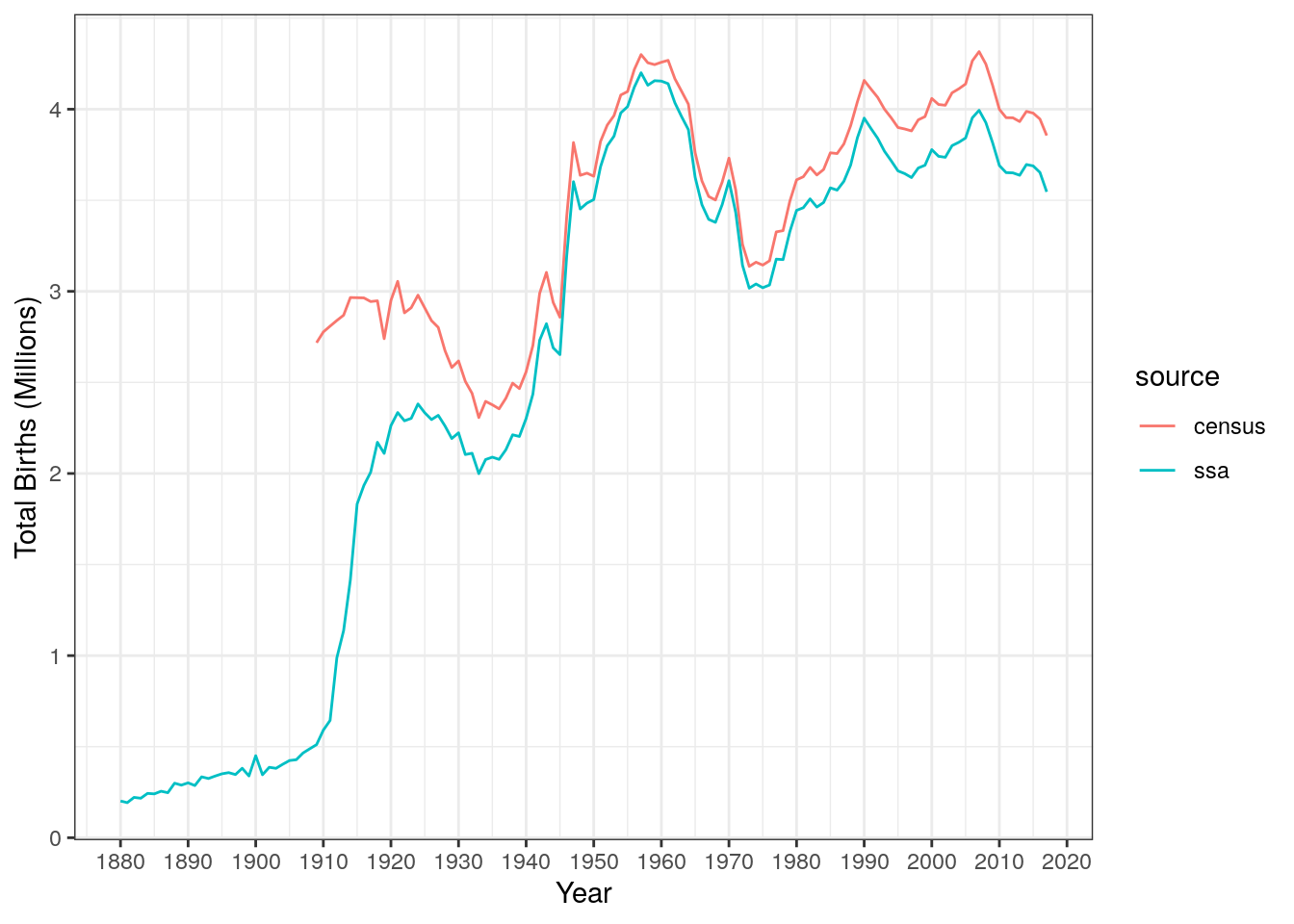# Reshaping data for fun, profit, and “tidyness”

## Goal

Become comfortable recognizing when reshaping data will make it better suited to the task at hand, and learn how to do so with the `pivot_longer()` and `pivot_wider()` verbs in the `tidyr` package (part of the all-powerful `tidyverse`).

Note: The reshaping performed by `pivot_longer()` and `pivot_wider()` was previously done by the verbs `gather()` and `spread()`, respectively. If you have the 1st edition of the textbook, you may have read about these. They still work, but have been superseded by the two `pivot_` functions, which are a bit simpler to use and also more flexible.

## The Data

Should we try to squeeze some more insight out of the `babynames` data? Let’s try to squeeze some more insight out of the `babynames` data. At least to start with.

Let’s make sure the relevant packages and datasets are loaded.

Code:

``````library(tidyverse)
library(babynames)
data(babynames)    ## SSA data
data(births)       ## Census data``````

### Some preparatory wrangling (and review of joins, etc.)

In the last lab, we joined the Social Security `babynames` data with the Census `births` data to produce a table that had two records of the total number of births in each year; one from each source.

Here’s the code we used to do it (below is the “full join” version).

Code:

``````census_births <- births
ssa_births <- babynames %>%
rename(num_births = n) %>%
group_by(year) %>%
summarize(
num_rows = n(),
births   = sum(num_births))
total_births <-
ssa_births %>%
full_join(census_births, by = "year")``````

To make sure it worked as expected let’s take a peek at a random sample of the joined data. Remeber that since a few years are only in one dataset or the other, there will be some missing values (`NA`).

Code:

``````total_births %>%
sample_n(size = 5)``````
``````## # A tibble: 5 x 4
##    year num_rows births.x births.y
##   <dbl>    <int>    <int>    <int>
## 1  1964    12396  3887800  4027490
## 2  1992    25427  3840196  4065014
## 3  1976    17391  3034949  3167788
## 4  1931     9297  2104071  2506000
## 5  1881     1935   192696       NA``````

The `births.x` and `births.y` variables are not very descriptive; also we don’t care so much about the `num_rows` variable, so let’s do some `select`ion (to remove `num_rows`) and `rename`ing (to replace the uninformative names with informative ones).

Code:

``````total_births <- total_births %>%
select(-num_rows) %>%
rename(
ssa    = births.x,
census = births.y)``````

Let’s look at a random sample from this modified data.

``total_births %>% sample_n(size = 5)``
``````## # A tibble: 5 x 3
##    year     ssa  census
##   <dbl>   <int>   <int>
## 1  1976 3034949 3167788
## 2  1989 3843559 4040958
## 3  2014 3696311 3988076
## 4  1999 3692537 3959417
## 5  1898  381458      NA``````

## Plotting birth counts by source

If we want to visualize the number of births over time from two different sources using two overlaid lines, we have to set the `y` aesthetic separately for each line.

Also, if we want to use a different color for each source, we have to specify them manually, line by line:

Code:

``````total_births %>%
ggplot(aes(x = year)) +
geom_line(aes(y = census), color = "blue", na.rm = TRUE) +
geom_line(aes(y = ssa), color = "orange", na.rm = TRUE) +
scale_x_continuous(
name   = "Year",
breaks = seq(1880,2020,by=10)) +
scale_y_continuous(
name   = "Total Births (Millions)",
breaks = seq(0,5000000,1000000),
labels = 0:5)``````We also don’t get a legend to tell us which source is which color. We could create this manually, but this is clunky and error-prone.

For a graph like this, we’d like to be able to create an aesthetic mapping between the `source` of the data and the `color` of the line. That mapping could then be used to automatically produce a legend. But `source` isn’t a variable in this data; it’s distinguished between variables, not between cases.

## Stacking data with the `pivot_longer()` function

Thinking about what the legend title and entries would be if we created one gives us a clue about what our dataset is missing: We need a variable called something like `source`, and a single variable to map to the \(y\)-axis, recording the number of births from the respective source.

We can use `pivot_longer()` for this, as follows:

Code:

``````births_long <- total_births %>%
pivot_longer(
cols      = c("census", "ssa"), # these are the columns we're "merging"
names_to  = "source",   # this is what we're going to call the new variable
values_to = "births",   # this is where the stacked values will go
)``````
``````## The earliest years are not in the census dataset
``````## # A tibble: 5 x 3
##    year source births
##   <dbl> <chr>   <int>
## 1  1880 census     NA
## 2  1880 ssa    201484
## 3  1881 census     NA
## 4  1881 ssa    192696
## 5  1882 census     NA``````

Having created the `source` variable and having merged all the counts into a single `births` variable, we can now create the line graph we want quite easily (and we get a legend automatically, since the color of the line now comes from a variable in the data table)

Code:

``````births_long %>%
ggplot(aes(x = year, y = births, color = source)) +
geom_line(na.rm = TRUE) +
scale_x_continuous(
name   = "Year",
breaks = seq(1880,2020,by=10)) +
scale_y_continuous(
name   = "Total Births (Millions)",
breaks = seq(0,5000000,1000000),
labels = 0:5)  ``````## The `pivot_wider()` function

Is the “long” format we’ve created “better” in an absolute sense? Well, it’s better for producing the line graph we wanted, but suppose we wanted to visualize the correlation between the sources with a scatterplot. For a plot like this, we want one axis to be the number of births according to the SSA, and the other axis to be the number of births according to the Census. This was easy in the original data:

Code:

``````total_births %>%
ggplot(aes(x = ssa, y = census)) +
geom_point(na.rm = TRUE) +
scale_x_continuous(
name   = "Births Recorded by the SSA (Millions)",
limits = c(0,5000000),
breaks = seq(0,5000000, by = 1000000),
labels = 0:5) +
scale_y_continuous(
name   = "Births Recorded by the Census (Millions)",
limits = c(2000000,5000000),
breaks = seq(2000000,5000000, by = 1000000),
labels = 2:5)``````